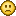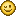### What's Max Value of Number atribute?

#### FortySe7en

Hey!
I'm doing a game in which I need to use really large numbers such as 2^64.
Problem is, pretty soon stencyl starts to change my number from normal format to a thing such as 8.796093022e+10

Question: what's the max number?
How can I move around that? (if possible)
That number will be the highscore, itunes accepts 2^64 as max value. I would like to get there with a normal number if possible.

#### captaincomic

Do you have a lot of zeros at the end of your high score? Then the easiest solution would be to store only the relevant digits and the number of zeros. For example, when the high score is 19 000 000 000 000 000, you store at as 19 and 15, and print it as 19 followed by 0 repeated 15 times.

#### FortySe7en

The score starts at 0.01 and doubles every time you kill an enemy.
It's a power of 2, so not really full of zerosProblem is, stencyl starts to use "e+1" way before I reach the max accepted score on itunes which is 2^64

#### captaincomic

That's gonna be difficult (at least if you want it to be precise.)
To get rid of the e+1 formatting you can use the following code (assuming _Score is the internal name of your score attribute)
Code: [Select]
`Std.string(_Score)`but if you try
Code: [Select]
`Std.string(Math.pow(2,64))`you'll get 18446744073709552000. While the result should be 18446744073709551616. That's because double precision numbers can only store ~16 significant digits. But maybe that's precise enough for a high score anyway#### mliga

Maybe you can split score to 2 (or more) number attributes, so say you have scoreLow to store the decimals  and digits up to 999999999.99 (something in the allowed range, and scoreHi to get highest  values. You can check overflow when increasing score,  and have scoreLow += remainder(  scoreLow / 1000000000) and overflow units added to scoreHi, the  può togheter the two in a text like scoreTxt = toText(scoreHi)+toText(scoreLow).
Sould be feasible with stencyl blocks.

#### FortySe7en

Thanks a lot guys! Good ideas.